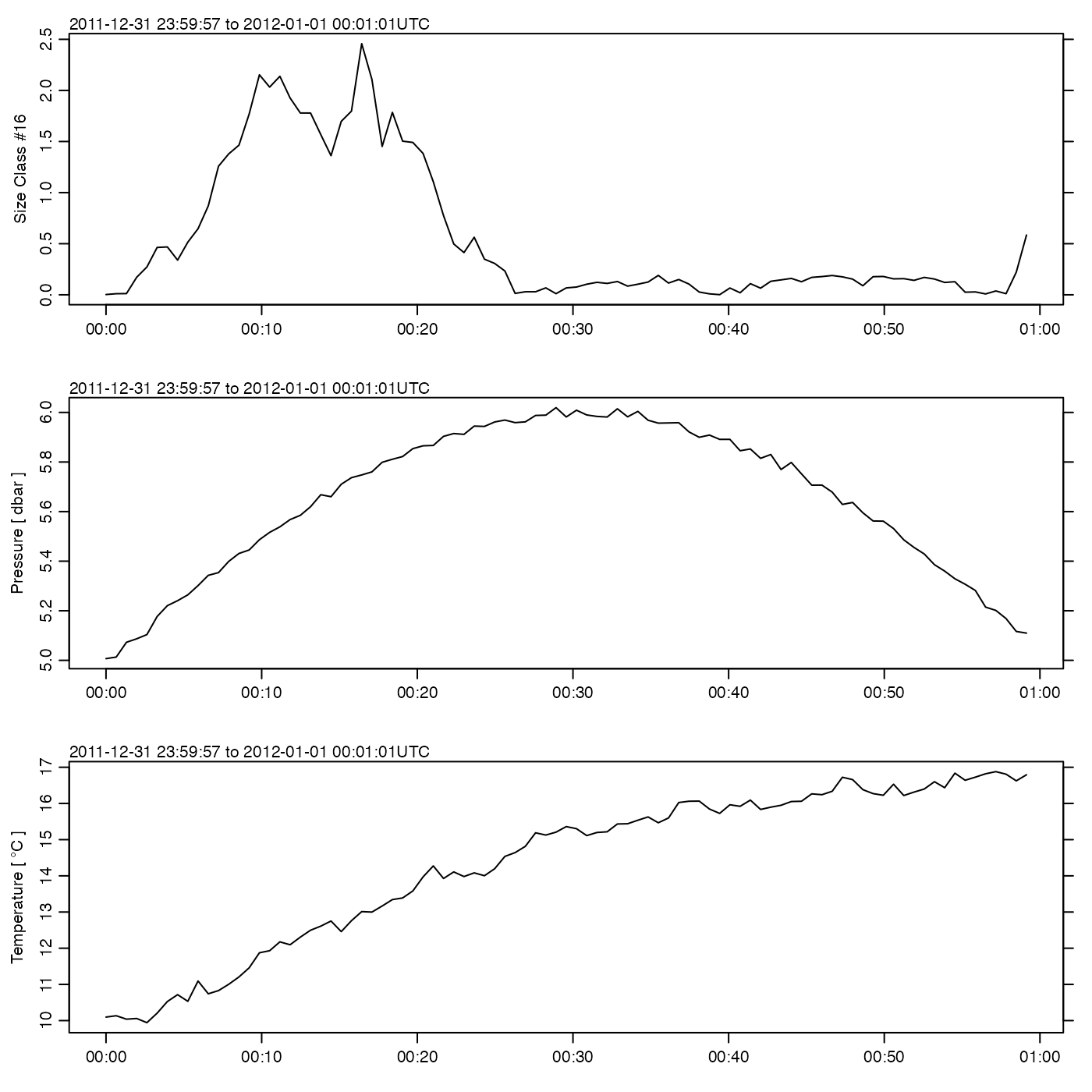Creates a multi-panel summary plot of data measured by LISST instrument.

# S4 method for lisst
plot(x, which = c(16, 37, 38), tformat, debug = getOption("oceDebug"), ...)

## Arguments

x

a lisst object.

which

list of desired plot types. These are graphed in panels running down from the top of the page. See “Details” for the meanings of various values of which.

tformat

optional argument passed to oce.plot.ts(), for plot types that call that function. (See strptime() for the format used.)

debug

a flag that turns on debugging. The value indicates the depth within the call stack to which debugging applies.

...

optional arguments passed to plotting functions.

## Details

The panels are controlled by the which argument, as follows.

• which=1 to 32, or which="C1" to "C32" for a time-series graph of the named column (a size class).

• which=33 or which="lts" for a time-series plot of laser transmission sensor.

• which=34 or which="voltage" for a time-series plot of instrument voltage.

• which=35 or which="aux" for a time-series plot of the external auxiliary input.

• which=36 or which="lrs" for a time-series plot of the laser reference sensor.

• which=37 or which="pressure" for a time-series plot of pressure.

• which=38 or which="temperature" for a time-series plot of temperature.

• which=41 or which="transmission" for a time-series plot of transmission, in percent.

• which=42 or which="beam" for a time-series plot of beam-C, in 1/metre.

The documentation for lisst explains the structure of lisst objects, and also outlines the other functions dealing with them.

Other functions that plot oce data: download.amsr(), plot,adp-method, plot,adv-method, plot,amsr-method, plot,argo-method, plot,bremen-method, plot,cm-method, plot,coastline-method, plot,ctd-method, plot,gps-method, plot,ladp-method, plot,landsat-method, plot,lobo-method, plot,met-method, plot,odf-method, plot,rsk-method, plot,satellite-method, plot,sealevel-method, plot,section-method, plot,tidem-method, plot,topo-method, plot,windrose-method, plot,xbt-method, plotProfile(), plotScan(), plotTS(), tidem-class

Other things related to lisst data: [[,lisst-method, [[<-,lisst-method, as.lisst(), lisst-class, read.lisst(), summary,lisst-method

Dan Kelley

## Examples

library(oce)
data(lisst)
plot(lisst)